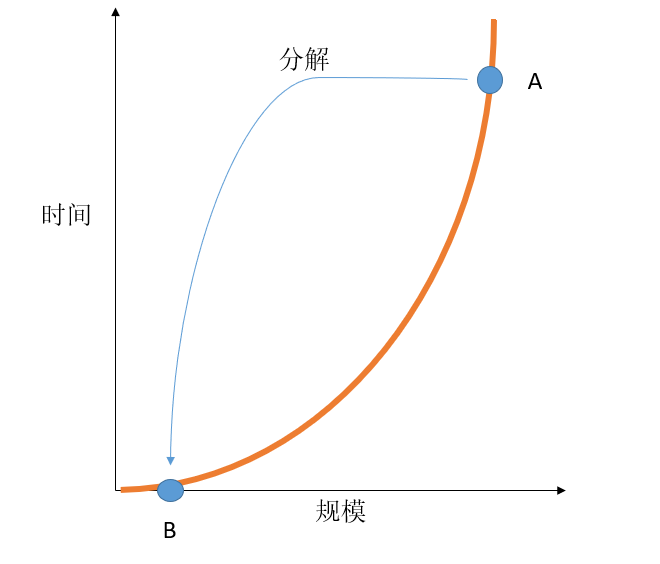归并排序、jensen不等式、非线性、深度学习

归并排序

def merge_sort(arr):
"""
input : unsorted array
output: sorted array in ascending order
"""
if len(arr) <= 1:
return arr
half = len(arr)//2
left = merge_sort(arr[0:half])
right = merge_sort(arr[half:])
left_tail ，right_tail = 0 ， 0
sorted_arr = []
while left_tail < len(left) and right_tail < len(right):
if left[left_tail] < right[right_tail]:
sorted_arr.append(left[left_tail])
left_tail += 1
else:
sorted_arr.append(right[right_tail])
right_tail += 1
if left_tail < len(left):
sorted_arr += left[left_tail:]
else:
sorted_arr += right[right_tail:]
return sorted_arr

非线性，Jensen 不等式$f(\sum_{i=1}^{N}\lambda_{i}x_{i}) < \sum_{i=1}^{N}\lambda_{i}f(x_{i})$

总结

posted @ 2019-09-16 13:26 kangheng 阅读(...) 评论(...) 编辑 收藏